﻿ Threshold Effect of State Budget Spending on Economic Growth: the Case of VietnamPublications are Open
Access in this journal
Article Versions
Export Article
• Normal Style
• MLA Style
• APA Style
• Chicago Style
Research Article
Open Access Peer-reviewed

### Threshold Effect of State Budget Spending on Economic Growth: the Case of Vietnam

Nguyen Thu Thuy , Ta Van Thang
American Journal of Modeling and Optimization. 2020, 8(1), 1-6. DOI: 10.12691/ajmo-8-1-1
Received April 19, 2020; Revised May 21, 2020; Accepted May 28, 2020

### Abstract

To the best of our knowledge, a very few studies have focused on the threshold effect of budget spending on economic growth in developing and developed countries. The purpose of this paper is to study the threshold effect of budget spending on economic growth in the Vietnam. Using a threshold analysis in the period over 1986-2018. The data were collected from Department of Statistics of Vietnam. Our results demonstrate that the optimal ratio of State budget expenditure to Vietnam's GDP is 24.67%. If this threshold is exceeded, the increase of the percentage ratio of State budget expenditure/GDP will have a negative impact on the speed of GDP growth.

### 1. Introduction

When we consider the economy as a whole made up of two parts, those are the state and the private sector. The private sector has now become the main sector for the production of material goods, while the state sector has played the role of providing public goods and services to ensure the smooth functioning of the private sector's economic activities effectively. When the scale of state budget spending is too small, the state does not have enough resources to spend on the task of providing public services, the economy thus operates less efficiently than it should, so economic growth also reached a low level. At this time, increasing the scale of budget spending will help the state have more conditions to build, consolidate and improve public services, making economic activities more efficient and leading to higher growth rates. However, if the scale of state budget spending is too large while the level of management is not high, the cash flow for public projects becomes less effective. At the same time, the funding for this budget is derived from the resources to produce private wealth through private revenue, which makes the economy inefficient. This is followed by a decrease in economic growth rate. At this time, increasing the scale of budget spending clearly makes the economic growth rate decrease.

The above analysis shows that, the scale of state budget expenditure exceeds a certain level, the economic growth rate slows down. There is a level of budget spending at which economic resources are most effectively allocated between public and private use. At this level, the economy will grow at the fastest sustained pace. Without exceeding this threshold, the size of budget spending and economic growth fluctuate in the same direction. Conversely, once the threshold is exceeded, the size of budget spending and economic growth will fluctuate in the opposite direction. Stemming from the goal of determining a reasonable scale of budget spending scale for Vietnam today, the authors have chosen the goal of determining a reasonable budget spending scale for the goal of economic growth in Vietnam.

The structure of the study is as follows. Section 2 discussed the literature review. Section 3 described the data, the research model, and methodology of this study. The empirical analysis is presented in section 4. Section 5 covers the conclusion.

### 2. Literature Review

The government's intervention in the economy through fiscal policy with tax tools and public expenditure has been widely recognized in the world for a long time. However, it was not until 1936 that with Keynes's aggregate demand model the effects of taxes and public spending on economic growth became clearer and more explicit. With this model, Keynes argued that the state could achieve its goal of creating effective aggregate demand through stimulus measures from public spending. Since then, Keynesian economists have always held that government spending - particularly spending through debt - can boost economic growth by increasing purchasing power (aggregate demand) of the economy. This theory was immediately responded by politicians because it gave them good reasons to spend. For a long time, researchers have tried to estimate the positive relationship between government spending and the level of output in the economy, but their estimation methods often faced many mistakes. The Keynesian theory ignores the fact that the government cannot pump purchasing power into the economy before reducing it through taxes and debt. As a result, Keynesian theory faced major challenges when the world economy fell into recession in the 1970s, and when there was an economic boom due to tax cuts combined with tightening spending in the 1980s.

By this time, a wave of opposition to Keynesian views had risen, and many economists believed that cutting the budget deficit was a miraculous remedy for economic growth. They argue that cutting government spending and thus cutting the budget deficit will reduce interest rates, increase investment, increase productivity and ultimately promote growth. This argument is grounded if the relationship between the above variables is close. However, there are many reasons to believe that the above hypothesis about the relationship between budget deficit, interest rate, investment and economic growth has been overemphasized. Specifically, the actual data of many countries in the world have shown that the budget deficit has a very small impact on interest rates, especially for open economies. The interest rate is determined on the international capital market where trillions of dollars are traded each day. Even a big change in the government's budget balance is unlikely to have a significant impact on interest rates. In addition, the main factor determining interest rates must be credit demand, which is why interest rates are often high during periods of strong growth. During these periods, credit demand is often high, and to earn profits financial institutions often impose high interest rates on loans to compensate for risks and inflation. In addition, when the other factors are the same, taxable loans often have a higher interest rate than non-taxable loans. This implies that the tax increase, while reducing the budget deficit, is more likely to raise interest rates and thus is unlikely to stimulate investment and economic growth. In line with these arguments, many empirical studies have been conducted and show that government spending cannot promote growth even with a negative impact on growth such as 6 and 7.

Hence, different theories use different arguments and therefore they do not give a clear answer on the relationship between government spending and economic growth. So, economic theory often does not explicitly indicate the impact of government spending on economic growth. However, most economists agree that there are certain cases where a reduction in government spending will promote economic growth, and there are also cases where an increase in government spending is beneficial for growth. Therefore, more sophisticated studies were conducted, the theory of public spending threshold was first developed by 9. His model reflects a more comprehensive relationship between the size of public expenditure and economic growth and it is widely used by economists in the world when studying the role of public spending. The Rahn curve implies that economic growth will be maximized when government spending is moderate and distributed to basic public goods such as infrastructure, property protection and law enforcement. However, public spending will be harmful to economic growth when it exceeds this limit, called the public spending threshold. The public spending threshold is the point where any increase in public spending below this value will have an impact on economic growth, while a larger increase will have a negative effect on economic growth. After that, 2, 3, 11 and 12 gave similar results.

However, so far, the role of public spending on economic growth remains a controversial topic. There have been a number of large-scale review works done to point out the consistency and differences through studies, and to explain those findings. The author in 8 have compiled 93 articles discussing the impact of fiscal policy on economic growth. Among these, 41 articles provide empirical evidence on the impact of the total size of public expenditure, with 29 percentage supporting the view that government expansion will curb growth, 17 percentage say that the opposite is true and more than half of the studies are inconclusive. The inconsistency in the results stems from some studies focusing on rich nations, while others focus on poor countries or put them all together. For studies that focus on a group of countries that share some characteristics, the findings appear to be more consistent. This is because the nature of public spending in each economy has its own characteristics, governed by certain characteristics (for example, level of development, political regime, institution, position or geography).

Most recently, 5 synthesized 87 empirical studies on the impact of the scale of public expenditure on economic growth. Using the general regression analysis method, the authors showed that the results found in the previous studies depended on the choice of variables, regression methods and national research group. Specifically, the negative effect was often found in developed country group studies, where the explanatory variable was the ratio of public expenditure to GDP and the dependent variable was the average GDP. When researching a developing country group, the estimation results were not statistically significant. And when all countries were included, the relationship was not statistically significant in the case of the explanatory variable as total government expenditure, but it was negative and significant in the case of the total expenditure variable for Government consumption was used. Here, total government expenditure was government expenditures that did not improve productivity.

Many other studies show the existence of thresholds of public expenditure scale such as 10 which showed that there was an Armey curve for Nigeria and the estimated optimal rate was found to be 19.81% of GDP when estimated for GDP per capita and optimal about 13% of GDP when estimates for real GDP; or 1 with their own research have shown that if public spending exceeds 30% of GDP, it will reduce economic growth and have no impact on the quality of life. It can be said that the above-mentioned studies provide a fairly clear picture of the research situation on the topic of relationship between public expenditure scale and economic growth.

### 3. Data and Methodology

3.1. Data

The data used in the study is sourced from the database of Department of Statistics (DOS) in Vietnam. Using a sample dataset during the period of time from 1986 to 2018 on a yearly basis, the data is analyzed using Stata 15 software. In this study, the data consist of two variables, those are ratio of state budget expenditure to GDP and GDP growth.

3.2. Research Model

This study focuses on analyzing the threshold of optimal state budget expenditure for economic growth, which is the percentage of total state budget expenditure to obtain Vietnam's optimal GDP, the point at which growth increases. The economy has the highest value and if the percentage of total state budget expenditure over GDP surpasses it, it will be inversely related to the GDP growth rate of Vietnam, thereby determining the total level of state budget expenditure to ensure fast and sustainable growth.

World studies on the threshold of state budget spending on economic growth have also been conducted in a number of countries and by a number of different methods. 13 showed that there existed a nonlinear relationship between government size and economic growth for ASEAN countries. The research model of this topic is also based on the two-region theoretical model of 4 and 9 and was extended by 14. Then, the estimation of threshold models, with the same independent variable as the economic growth rate with different independent combination of variables, and using SSR, AIC, BIC model selection criteria and HQIC to make a choice of the most appropriate model, corresponding to the most appropriate model which will be the most appropriate threshold of the ratio of state budget expenditure to GDP. In addition, with the independent variables included in the regression model, there are lag variables of the independent variables and threshold variables, rather than fixing a fixed form of function. This has not yet done in 13. That is, the implementation method has improvements compared to 13. We will build a series of models as follows to study the effect of budget spending on GDP growth.(3.1)

Where:

GDP_growth is real GDP growth (dependent variable);

GSt is an explanatory variable and a threshold variable representing state budget expenditure, calculated as the ratio of state budget expenditure to GDP (compared to 2010 price). This is also the threshold variable.

GOVt = Gt * GSt, Gt is the growth of state budget expenditure, GSt is the above threshold variable.

INVt is an independent variable, representing the ratio of non-state investment to total GDP.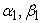are the estimated coefficients on each domain.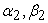are regression coefficients describing the effect of state budget expenditure on real GDP growth rate in each region.are regression coefficients that describe the effect of GOVt on real GDP growth rates across each region.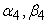are the regression coefficients that describe the effect of INVt on real GDP growth rates across each region.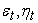is the estimated error of the model, which is independent, identically distributed, with a 0 expectation and a constant variance.

t is the year index, showing the differences between the years studied.

### 4. Results of Economic Modeling

4.1. Descriptive Statistics

Statistical description of the variables studied in the period from 1986 to 2018 is shown in Table 2 below.

Table 2 shows that the average real growth rate was 6.7%, with the highest rate of 9.54% in 1995, the lowest at 3.58% in 1987. The average budget expenditure in the research period 26.47%, with the highest budget expenditure being 32.03%; in 2007, the highest budget expenditure was 15.75% in 1991.

For visualization, we can look at the graphs of GDP_GROWTH, GS, GOV and INV as in Figure 1.

4.2. Estimation Models

The regression results of the dependent variable model GDP_GROWTH with three independent variables GS, GOV, INV, with the threshold variable being the GS variable, obtained a threshold model as shown in Table 3.

• Figure 1. Graph of variables

The estimated threshold of state budget expenditure is 24.67% of the sample divided into 2 regions. Domain 1 corresponds to parts of the sample where state budget spending is less than or equal to 24.67%. Region 2 corresponds to parts of the sample where state budget expenditure is greater than 24.67%.

However, in Table 3 the estimated coefficients found for the GS threshold variable are negative and are not statistically significant at the 5% significance level. This leads us to estimate a new threshold model whose dependent variable is GDP_GROWTH according to two independent variables GS, GOV, with the threshold variable GS, to verify whether there is a GS threshold for the GDP_GROWTH variable. The results are as in Table 4.

The estimated threshold of state budget expenditure in this model is still 24.67% dividing the research sample into 2 regions. Domain 1 corresponds to parts of the sample where state budget spending is less than or equal to 24.67%. Region 2 corresponds to parts of the sample where state budget expenditure is greater than 24.67%.

The results show that, in region 1, that is, the state budget expenditure is below 24.67%, then state budget expenditure has a positive effect on economic growth, expressed in the regression coefficient of state budget expenditure variable is 0.3304, statistically significant at the 5% significance level. GOV also has a positive effect at the 5% statistical level on economic growth, since the probability value is 0.003 which is lower than 0.05, this effect is reflected in the regression coefficient of 0.0026. In domain 2, that is, at the level of state budget expenditure above 24.67%, state budget expenditure has the opposite effect on economic growth, reflected in the regression coefficient of the state budget expenditure variable is -0.4601, statistically significant at the 5% significance level. GOV in this case also has a negative impact on economic growth, shown in the regression coefficient of -0.0020 with statistical significance at 10%.

The results of this model confirm the inverse U-shaped relationship between economic growth and the scale of budget expenditure in Vietnam during 1986-2018, the optimal threshold of budget spending on economic growth in the period. The review period is 24.67%. However, not all spending or investment can generate revenue at that time, their effectiveness may be delayed by a few lags.

After a series of models with changes in threshold variables and independent variables with additional lags, the best threshold regression model was chosen to determine the optimal threshold of budget spending on economic growth. is a model with dependent variable GDP_GROWTH and two independent variables GS, GOV and GS are threshold variables, as shown in Table 4 aboves.

Before using this threshold model for analysis, we need to perform a test of whether the received threshold is statistically significant. Testing is done by Bootstrap presented in the following Table 5.

Table 5 presents the test statistic according to the probability of the Bootstrap method of threshold values of state budget expenditure to total GDP. With a 5% significance level of the statistical value, Bootstrap results support the threshold effect. Mean threshold value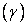have values consistent with the actual valueFrom this, we can use this model to analyze the threshold impact of state budget expenditure on economic growth:(4.1)

### 5. Conclusion

The model results clearly illustrate the expected nonlinear relationship between economic growth and the scale of budget expenditure in Vietnam during 1986-2018. It is expected that, below the threshold, state budget spending has the same directional effect on economic growth, and above the threshold, state budget spending has the opposite effect on economic growth. And the optimal threshold of budget expenditure for economic growth in the period considered is 24.67%. The optimal threshold of budget spending in relation to economic growth is interpreted as the point where any increase in budget spending below this value will have a positive effect, while a larger amount will have a negative effect on economic growth.

The estimated threshold of 24.67% divides the sample into 2 regions. Domain 1 corresponds to parts of the sample where state budget spending is less than or equal to 24.67%. Region 2 corresponds to parts of the sample where state budget expenditure is greater than 24.67%. GOV in this case also has a negative impact on economic growth, shown in the regression coefficient of -0.0020 with statistical significance at 10%. This indicates that when the GOV increases by 1%, the real GDP growth rate decreases by 0.0020%. The ratio of total state budget expenditure to Vietnam's optimal GDP is 24.67%, if this threshold is exceeded, the increase of the percentage of total state budget expenditure/GDP will have a negative impact on the speed of GDP growth. Thus, the model results show that the ratio of total optimal state budget expenditure on GDP in Vietnam in the period of 1986-2018 is 24.67%.

### References

  Afonso, A., Schuknecht, L. & Tanzi, V. “Public Sector Efficiency: An International Comparison”, Public Choice, 123 (3-4), 321-347. 2005. In article View Article  Armey, D. and Armey R., The Freedom Revolution: The New Republican House Majority Leaders Tells why Big Governments failed, Why Freedom works, and How we will rebuild America, Wasgington D.C.; Regnery Publishing Inc. 1995. In article  Barro, Robert J. (1990) “Government Spending in a Simple Model of Endogenous Growth”, Journal of Political Economy 98, S (5): 103-125. In article View Article  Chen, S. T. & Lee, C. C., “Government size and economic growth in Taiwan: A threshold regression approach”, Journal of Policy Modeling, 27(9). 1051-1066. 2005. In article View Article  Churchill, S. A., Yew, S. L. & Ugur, M. Effects of Government Education and Health Expenditures on Economic Growth: A Meta-Analysis, Greenwich Political Economy Research Center, Greenwich University, Greenwich. 2015. In article  Kormendi, R. C. & Meguire, P. G., “Macroeconomic determinants of growth: Cross-country evidence”, Journal of Monetary Economics, Elsevier, 16(2), 141-163, Sep.1985. In article View Article  Landau, D. “Government expenditure and economic growth: a cross-country study”, Southern Economic Journal. Jan.1983. In article View Article  Nijkamp, P. & Poot, J. “Meta-analysis of the effect of fiscal policies on long-run growth”, European Journal of Political Economy, 20, 91-124. 2004. In article View Article  Rahn, “Government Size and Economic Growth: a New Framework and Some/Evidence from Cross-Section and Time-Series Data”, The American Economic Review, Mar.1986. In article  Santos, A. R. “Does Optimal Government Size Exist for Developing Economies? The Case of Nigeria”, AAU Annals of Accounting, Educational & Social Research, 1(1): 15-33, Jun.2014. In article  Scully, G., “What is the optimal Size of government in the US?”, National Center for Policy Analysis, Policy Report No. 188. 1994. In article  Scully, G., “The Growth-Maximizing Tax-rate”, Pacific Economic Review, 5(1), 2000. In article View Article  Thanh, S. D. and Hoai, B. M. “The threshold of government size and economic growth for ASEAN countries: an analysis of the smooth transition regression model”, Southeast Asian Journal of Economics, 3(1), 103-124, Jun.2015. In article  Odawara, R. “A threshold approach to measuring the impact of government size on economic growth”, The George Washington University. 2010. Retrieved 10 May 2014 from http://www.thebrokenwindow.net/papers/J/JobPaper_Odawara.pdf. In article

Published with license by Science and Education Publishing, Copyright © 2020 Nguyen Thu Thuy and Ta Van ThangThis work is licensed under a Creative Commons Attribution 4.0 International License. To view a copy of this license, visit http://creativecommons.org/licenses/by/4.0/